# Power Diodes: What Are They? (Characteristics & Softness Factor)

Contents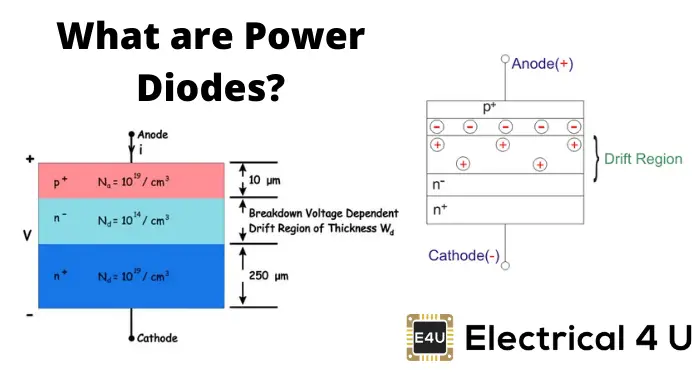## What is a Power Diode?

A power diode is a type of diode that is commonly used in power electronics circuits. Just like a regular diode, a power diode has two-terminals and conducts current in one direction. A power diode varies in construction from a standard diode to enable this higher current rating.

To better understand how a power diode differs from a regular diode, let’s revisit how a standard diode works.

Diodes are the simplest semiconductor device having only two layers, two terminals, and one junction.

The ordinary signal diodes have a junction formed by p type semiconductor and n type semiconductor, the lead joining p-type is called the anode and the other side lead joining the n-type is called the cathode.

The figure below depicts the structure of an ordinary diode and its symbol.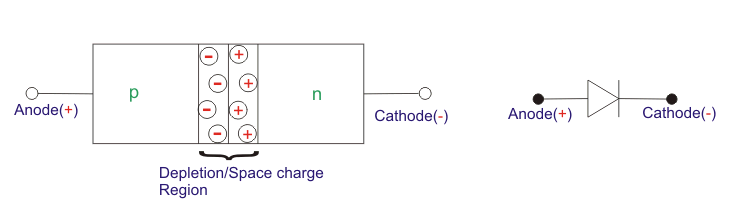Power diodes are also similar to regular diodes, although they vary slightly in their construction.

In regular diodes (also known as “signal diode”), the doping level of both P and N sides is the same and hence we get a PN junction, but in power diodes, we have a junction formed between a heavily doped P+ and a lightly doped N the layer which is epitaxially grown on a heavily doped N+ layer. Hence the structure looks as shown in the figure below.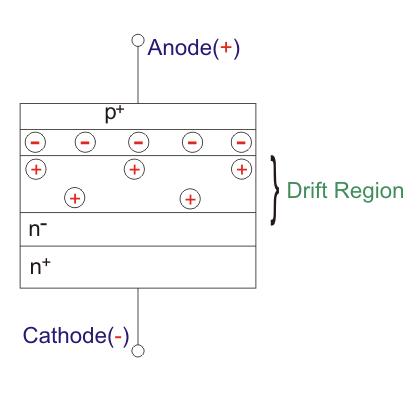The N layer is the key feature of the power diode which makes it suitable for high power applications. This layer is very lightly doped, almost intrinsic and hence the device is also known as a PIN diode, where i stands for intrinsic.

As we can see in the figure above that the net charge neutrality of the space charge region is still maintained as was the case in the signal diode but the thickness of the space charge region is quite high and deeply penetrated into the N region.This is due to its light doping concentration, as we know that the thickness of the space charge region increases with a decrease in doping concentration.

This increased thickness of the depletion region or the space charge region helps the diode to block larger reverse-biased voltage and hence have a greater breakdown voltage.

However, adding this N layer significantly increases the ohmic resistance of the diode leading to more heat generation during the forwarding conduction state. Hence power diodes come with various mountings for proper heat dissipation.

## V-I Characteristics of Power Diodes

The figure below shows the v-i characteristics of a power diode which is almost similar to that of a signal diode.In signal diodes for forward, a biased region the current increases exponentially however in power diodes high forward current leads to high ohmic drop which dominates the exponential growth and the curve increases almost linearly.

The maximum reverse voltage that the diode can withstand is depicted by VRRM, i.e. peak reverse repetitive voltage.

Above this voltage, the reverse current becomes very high abruptly and as the diode is not designed to dissipate such a high amount of heat, it may get destroyed. This voltage may also be called peak inverse voltage (PIV).

## Reverse Recovery Characteristics of Power Diodes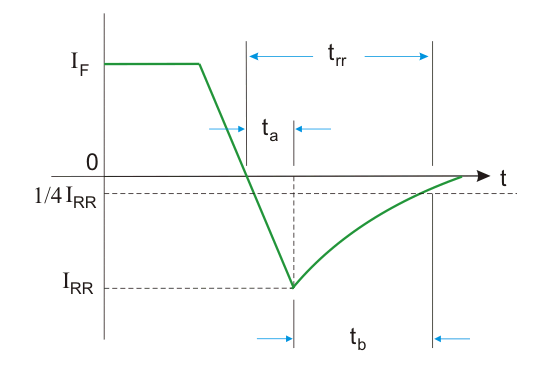The figure depicts the reverse recovery characteristic of a power diode. Whenever the diode is switched off the current decays from IF to zero and further continues in reverse direction owing to the charges stored in the space charge region and the semiconductor region.

This reverse current attains a peak IRR and again starts approaching zero value and finally, the diode is off after time trr.

This time is defined as reverse recovery time and is defined as the time between the instant forward current reaches zero and the instant the reverse current decays to 25% of IRR. After this time the diode is said to attain its reverse blocking capability.

From the figure above, we see that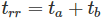ta → time when charge from depletion region is removed
tb → time when charge from semiconductor region is removed
Also from the figure, we can say that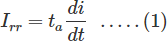Where,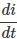is the rate of change of reverse current.
The area bounded by the triangular region in the above figure represents the total charge stored or reverse recovery charge, QR. Hence we can write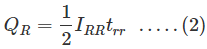Now, for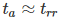, putting in eq.1 and combining with eq.2, we getPutting eq.3 in eq.1 for, we get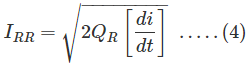From eq. 3 and 4 we can see that trr and IRR depend on QR which in turn depends upon the initial forward diode current IF.

Another interesting parameter is defined for power diodes from its turn-off characteristics known as Softness Factor (S-factor) defined as the ratio of times tb and ta.
Hence,If a diode has S-factor equals to unity it is known as a soft-recovery diode and for S-factor less that unity it is known as fast or snappy-recovery diodes.

S-factor indirectly indicates the voltage transient that occurs upon the turn off of the diode. Low S-factor implies high transient overvoltage while high S-factor implies low oscillatory reverse voltage.

The total power loss during turn off is the product of diode current and voltage during trr. Most of the power loss occurs during tb.

In a typical datasheet of power diodes the most important parameters given are IF avg, IF RMS, VRRM, I2t rating, junction temp TJ, trr, S-factor, IRR. Apart from these many other parameters and graphs are also provided.

The power diodes can be classified into the following categories, summarized in the table below, as per their properties:

 Type Voltage ratings (VRRM) Current ratings (IF) Reverse recovery time (trr) Applications Remarks General Purpose Diodes 50-5000 V 1A to several thousand Amps ~25µs UPS, battery chargers, welding, traction, etc. – Fast Recovery Diode 50-3000 V 1A to several thousand Amps <5µs SMPS, commutation circuits, choppers, induction heating Doping is done using platinum or gold Schottky Diodes Up to 100V 1-300 A ~ns Very high frequency switching power supplies and instrumentation Metal-semiconductor junction, usually Al-Si(n-type), majority carrier device, hence very low turn off time

Want To Learn Faster? 🎓
Get electrical articles delivered to your inbox every week.
No credit card required—it’s 100% free.